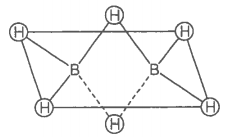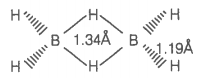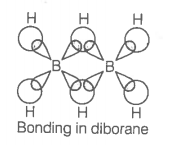Which of the following statements are correct?

(Answer on the basis of the given figure.)a. The two bridged hydrogen atoms and the two boron atoms lie in one plane

b.  Out of six B-H bonds, two bonds can be described in terms of 3-center-2-electron bonds

c.  Out of six B-H bonds, four B-H bonds can be described in terms of 3 center 2 electron bonds

d.  The four-terminal B-H bonds are two center-two electron angular bonds

Choose the correct option

1. (a, b, c)
2. (b, c, d)
3. (a, c, d)
4. (a, b, d)

Hint: Bridge bonds are 3center 2-electrons system

The bonding and structure of the boranes are of great interest. They are different from all other hydrides as they are electron deficient.
In diborane, there are 12 valence electrons, three from each B-atom and six from H-atoms.

Electron structure is shown in the figure:The two bridging H-atoms are in a plane perpendicular to the rest of the molecule and prevent rotation between the two B-atoms.

The terminal B-H distances are the same as the bond lengths measured in non-electron deficient compounds. These are assumed to be normal covalent bonds, with two electrons shared between two atoms. We can describe these bonds are two centre two electron bond (2c-2e).

Obviously, they are abnormal bonds as the two bridges involve only one electron each from one of the boron atoms and hydrogen atoms, making a total of four electrons.

According to molecular orbital theory, each B-atom uses sp3-hybrid orbitals for bonding. Out of the four sp3-hybrid orbitals on each B-atom, one is without an electron shown in broken lines.

The terminal B-H bonds are normal 2 centre-2 electron bonds but the two bridge bonds are 3 centre-2 electron bonds. The 3-centre-2 electron bridge bonds are also called banana bonds.